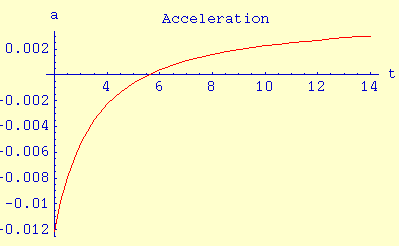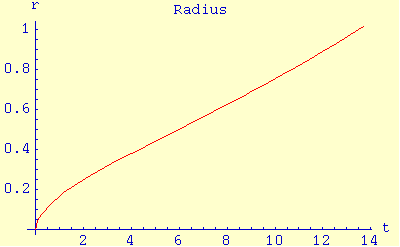Home | Science | * Dark Energy | * The Physics of Dark Energy 01. Differential Equations 02. Classical Cosmology 03. Relativistic Cosmology 04. Big Bang Cosmology 05. Dark Energy Observed 06. A Dark Energy Model 07. Discussion and Conclusions 08. Space Applet 09. Space Applet Java ListingShare This Page
The Physics of Dark Energy
An exploration of a recent discovery in cosmology.

(double-click any word to see its definition)

A Dark Energy Model

Because the previously described equation set has no closed-form solution, and because a number of the properties of "dark energy" are at present only estimated, I have written a simple empirical equation meant to show the probable evolution of the universe based on what we know at this point. This equation is in good agreement with observations, but it doesn't pretend to address underlying physical causes. Here it is:

 (9)  f(t) = 1 t1/1.8 t2.146 150

The argument to this equation is billions of years (reminding my readers that I am using the U.S. convention for "billions", that is, thousand millions or 109). The result of the equation is the overall size of the universe.

This equation meets a number of relevant criteria. First, it represents an abbreviated numerical solution to the differential equation set previously described (equations 6,7, and 8 on this page). Next, it switches from deceleration to acceleration at about six billion years, in accordance with present observations. Finally, it produces a result normalized to 1.0 for the present estimated age of the universe (13.7 billion years). This normalization allows us to easily compare past and future sizes of the universe with the present.

The basic idea of the new dark-energy based cosmological model is that the universe began with a relatively high radial velocity, gradually decelerated through mutual gravitational attraction until about six billion years, then deceleration changed to acceleration as dark energy's repulsive force began to overwhelm mutual gravitation. In the future, if prevailing assumptions about dark energy turn out to be correct, the acceleration is expected to increase without bound, leading to a very large universe with steadily decreasing density of mass-energy — that is to say, a cold, dark future.

Here are some graphs derived from this equation, with explanatory notes:

 Description Graphic Acceleration history, 2 to 14 billion years. Note that the negative acceleration of gravitation prevailed until about six billion years, after which dark energy produced a switch to positive acceleration.Velocity history, 0 to 14 billion years (some values off scale near time = 0). Note that the velocity of cosmological expansion, very large near the time of the Big Bang, reached a minimum at about six billion years, after which dark energy caused it to increase (and it will continue to do so).Cosmological radius, short-term, 0 to 14 billion years, values normalized to 1.0 for the present age of the universe (estimated to be 13.7 billion years). Note that the expansion follows a classic gravitational deceleration pattern until about six billion years, and that it has now switched to a pattern of exponential increase.Cosmological radius, long-term, 0 to 40 billion years. This graph clearly shows the expected long-term exponential pattern of expansion, eventually converging with t2. Note also that the universe will have a radius about four times its present value at 40 billion years, corresponding to a mass-energy density of 1/64th its present value. The implications of this change in mass-energy density should be obvious — no more galactic collisions with attendant conditions favorable to stellar birth, a consequent end to the formation of new stars, and an overall temperature drop for a multitude of reasons. A cold, dark future for the universe.I want to emphasize that this equation is empirical and approximate, but that it roughly corresponds with conclusions drawn from observation.

 Home | Science | * Dark Energy | * The Physics of Dark Energy 01. Differential Equations 02. Classical Cosmology 03. Relativistic Cosmology 04. Big Bang Cosmology 05. Dark Energy Observed 06. A Dark Energy Model 07. Discussion and Conclusions 08. Space Applet 09. Space Applet Java ListingShare This Page